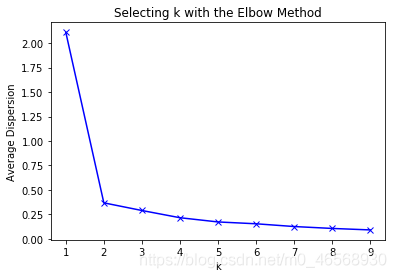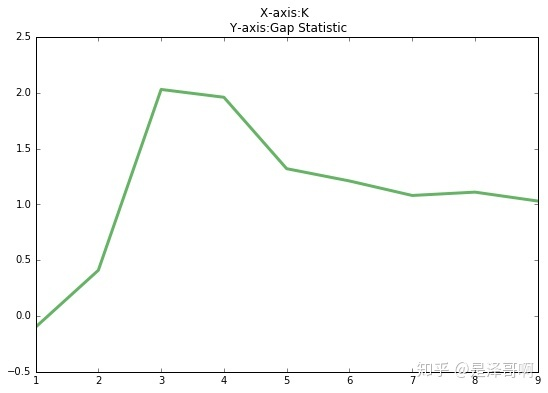K-means## 算法流程

1. 随机选择 $k$ 个样本作为初始化聚类中心：$a_1, a_2, \dots, a_k$ ；
2. 针对数据集每个样本 $x_i$ ，计算其到每个聚类中心的距离并将其分到距离最小的聚类中心所对应的类中；
3. 针对每个类别 $a_j$ ，重新计算它的聚类中心：$a_j = \frac{1}{|c_j|} \sum_{x \in c_j} x$ （即该类所有样本的质心）；
4. 重复2-3步，直到达到某个终止条件（迭代次数、最小误差变化等）。

## 优缺点

#### 优点

• 容易理解，聚类效果不错，虽然是局部最优， 往往局部最优已经足够
• 处理大数据集的时候，该算法可以保证较好的伸缩性
• 算法复杂性低

#### 缺点

• $K$ 需要认为设定，不同的取值对最终聚类效果影响非常大
• 对初始簇类中心敏感，不同的初始取值对最终聚类效果影响非常大
• 对异常值敏感
• 不适合离散的分类、样本类别不平衡的分类、非凸形状的分类

## 如何选择 $K$ 值？

#### 手肘法#### Gap statistic## 如何初始化聚类中心？

1. 随机选择一个中心点 $a_1$ ；

2. 对于数据集中的每一个点 $x_i$ ，计算它与之前 $n$ 个聚类中心最远的距离 $D(x_i)$ ，并以 $\frac{D(x_i)^2}{\sum_{j=1}^n D(x_j)^2}$ 的概率选择作为新中心点 $a_i$ ；

3. 重复2步骤，直到选出 $K$ 个聚类中心。